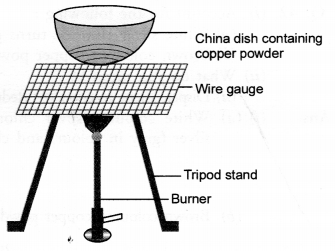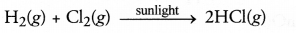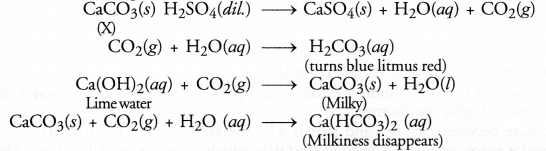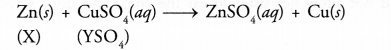## HOTS Questions for Class 10 Science Chapter 1 Chemical Reactions and Equations

These Solutions are part of HOTS Questions for Class 10 Science. Here we have given HOTS Questions for Class 10 Science Chapter 1 Chemical Reactions and Equations

Question 1.
Study the given diagram and answer the following questions :
(a) Write the chemical reaction involved in the process.
(b) Mention the colour of :

1. copper powder and
2. the substance formed after heating it.

(c) How can we reverse the above reaction ? Write the equation for the reverse reaction and state the substance that undergoes oxidation and the substance that undergoes reduction.
(CBSE 2015)(a) Copper powder upon heating reacts with oxygen (present in air) to form cupric oxide or copper (II) oxide.(b)

1. Copper powder is brownish in colour.
2. The substance formed after heating is cupric oxide. It has black colour.

(c) The reaction can be reversed by heating cupric oxide in a current of hydrogen.
CuO(s) + H2(g) ———–> Cu (s) + H2O(g)
In this reaction, H2 undergoes oxidation to H2O and CuO is reduced to Cu.

More Resources

Question 2.
The gases hydrogen and chlorine do not react with each other even if kept together for a long time. However, in the presence of sun light, they readily combine. What does actually happen ?
We know that in chemical reactions, energy is needed to break the bonds present in the reacting molecules so that they may combine to form the products. In the present case, sun light is the source of energy in the form of photons. The energy made available helps in breaking the bonds present in the reactant molecules and the chemical reaction leading to hydrogen chloride gas takes place.Question 3.
A water insoluble substance ‘X’ on reacting with dilute H2SO4 released a colourless and odourless gas accompanied by brisk effervescence. When the gas was passed through water, the solution obtained turned blue litmus red. On bubbling the gas through lime water, it initially became milky and the milkiness disappeared when the gas was passed in excess. Identify the substance ‘X’ and write the chemical equations of the reactions involved.
The water in soluble substance ‘X’ is most probably some metal carbonate (CaCO3). The chemical equations that are involved are given.Question 4.
(a) Based on the reactions given below, arrange the metals involved in these reactions in decreasing order of reactivity. Give suitable explanation.

1. Zn + CuSO4 ———> ZnSO4 + Cu
2. Cu + 2AgNO3 ———> Cu(NO3)2 + 2Ag
3. Zn + FeSO4 ———-> ZnSO4 + Fe
4. Fe + CuSO4 ———-> FeSO4 + Cu

(b) What is the nature of the reactions ?
(a)

1. Since Zn displaces Cu from CuSO4 solution, it is more reactive than Cu.
2. Since Cu displaces Ag from AgNO3 solution, it is more reactive than Ag.
3. Since Zn displaces Fe from FeSO4 solution, it is more reactive than Fe.
4. Since Fe displaces Cu from CuSO4 solution, it is more reactive than Cu.
The decreasing order of reactivity of the metals is :
Zn > Fe> Cu> Ag

(b) All these reactions are the examples of displacement reactions.

Question 5.
A, B and C are three elements which undergo chemical reactions according to following equations.
A2O3 + 2B ———-> B2O3 + 2A
3CSO4 + 2B ———-> B2(SO4)3 + 3C
3CO + 2A ———> A2O3 + 3C
(a) Which element is the most reactive ?
(b) Which element is the least reactive ?
(a) The most reactive element is ‘B’ as it has displaced both A’ and ‘C’ from their compounds.
(b) The least reactive element is ‘C’ as it has been replaced by both A’ and ‘B’.

Question 6.
You are given the following materials

1. Marble chips
2. Dilute hydrochloric acid
3. Zinc granules

Identify the type of reaction when marble chips and zinc granules are added separately to acid taken in two tubes. Write chemical equations in each case.

1. Marble chips react with dilute hydrochloric acid to form calcium chloride and carbonic acid. The acid changes to CO2 and H2O. It is a double displacement reaction.
CaCO3(s) + 2HCl (aq) ———–> CaCl2(aq) + H2CO3(aq) (CO2+H2O)
2. Zinc granules react with hydrochloric acid to form zinc chloride accompanied by hydrogen gas. It is a displacement reaction.
Zn(r) + 2HCl(aq) ———–> ZnCl2(aq) + H2(g)

Question 7.
A strip of a metal X is immersed in the aqueous solution of salt YSO4 blue in colour. After sometime, a layer the metal Y from the salt solution is deposited on the strip of the metal X. Whereas the metal X is used for galvanisation, the metal Y is employed in making electric cables.

1. Predict the metal X.
2. What could be the metal Y ?
3. Can yon name the salt YSO4 ?
4. What is the nature of the chemical reaction taking place ?